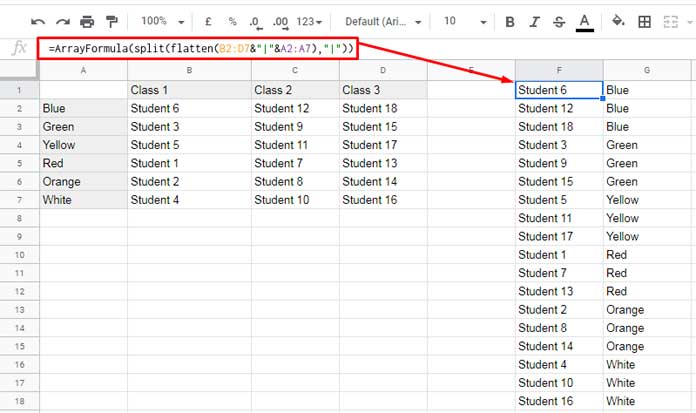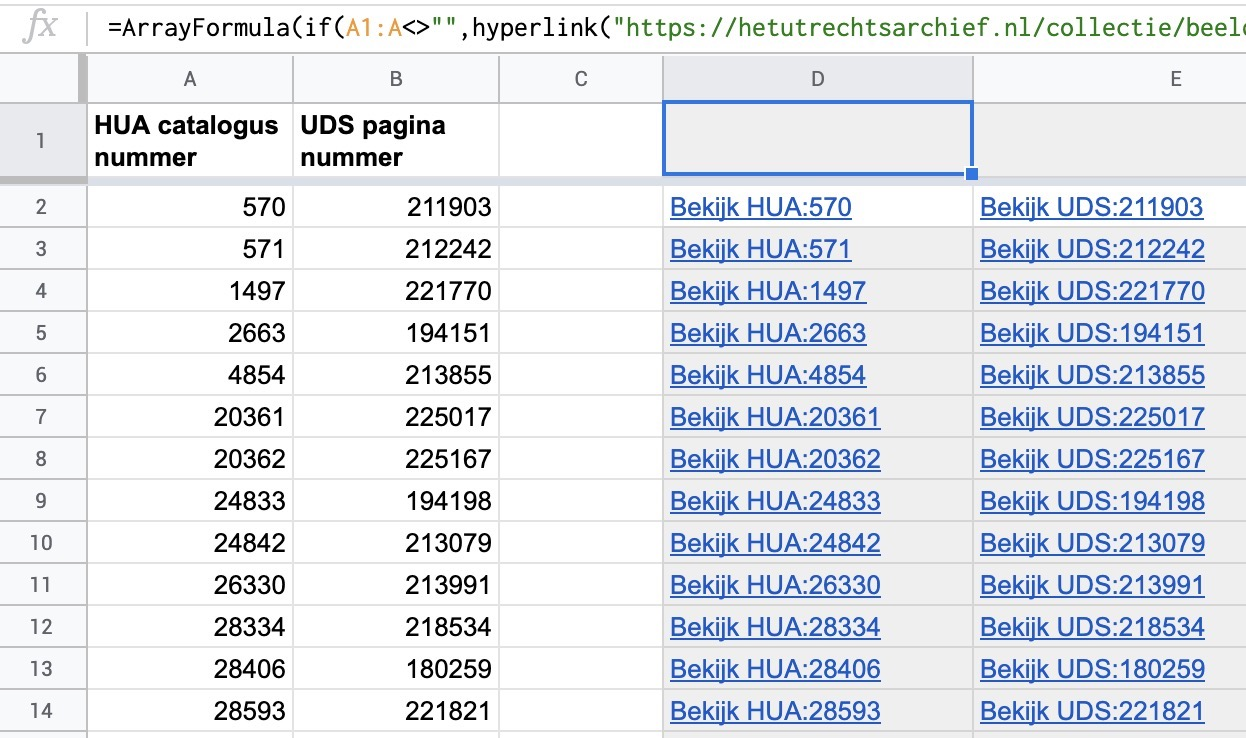# sheets

## make column with unique values from a matrix

``=SORT(UNIQUE(FLATTEN(A2:E)))``

## replace

``lower(REGEXREPLACE(REGEXREPLACE( REGEXREPLACE(REGEXREPLACE(Trefwoorden!C2:C; "[–’+=&?|,\.() ""\$/':;]"; "-") ;"-+";"-"); "[^a-zA-Z0-9\-]";"");"^-|-\$"; ""))``

## Vlookup with Search Key in Multiple Column Range``=ArrayFormula(split(flatten(B2:D7&"|"&A2:A7);"|"))``

## transpose comma separated values in multiple rows to one long list of items

``=transpose(split(join(",";D:D);","))``

## shortcut for new sheet

http://sheets.new

``````'place this in the header cell above a column, that way you will still be able to sort the sheet